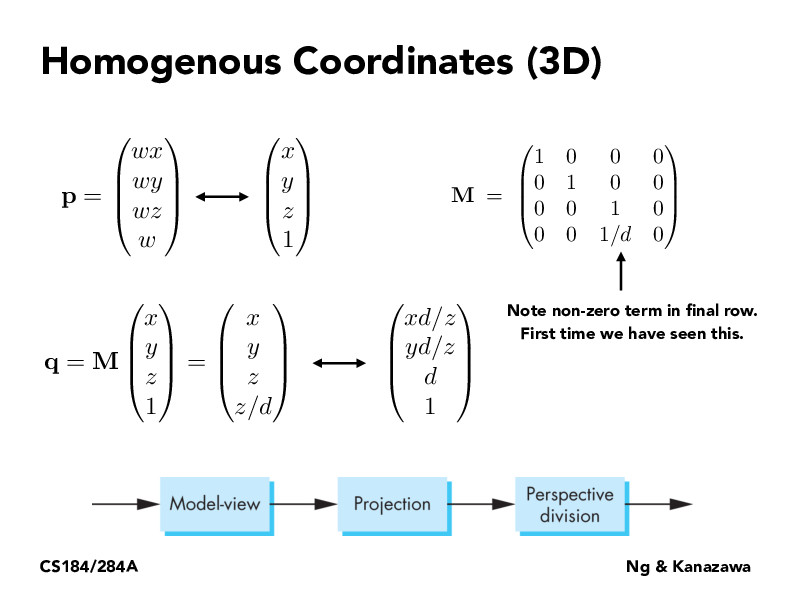Lecture 4: Transforms (87)c4554ndr4

Why are we scaling the vector water multiplying it by M? Is this just so M looks nicer? Or is this the only way to write it in homogenous coordinates?SainanChen

I am confused... If we can get (xd/z yd/z d 1)T from (x y z z/d)T, why couldn't we get (xd/z yd/z d)T directly from (x y z)T by multiplying every element by d/z but using matrix multiplication?wcyjames

@SainanChen: I think there is no easy way to multiply z by d/z directly, since d/z is a term containing z itself. But using homogenous coordinates solves this problem, and many other operations can also be done by matrix multiplications too!ziyaointl

@wcyjames Exactly! This is so that $M$ doesn't have to change with respect to every possible $z$.

You must be enrolled in the course to comment